# Influence of Hall and ion-slip currents on unsteady MHD free convective flow of a rotating fluid past an oscillating vertical plate

Influence of Hall and ion-slip currents on unsteady MHD free convective flow of a rotating fluid past an oscillating vertical plate

Jitendra K. Singh Pratima Rohidas Naveen Joshi Shaikh G. Begum

Department of Mathematics, V.S.K. University, Ballari-583 105, Karnataka, INDIA

Corresponding Author Email:
s.jitendrak@yahoo.com
Page:
37-52
|
DOI:
https://doi.org/10.18280/ijht.350106
|
Accepted:
|
Published:
31 March 2017
| Citation

OPEN ACCESS

Abstract:

A mathematical analysis of unsteady MHD free convective flow of an incompressible, chemically reacting, electrically and thermally conducting fluid past an oscillating vertical plate embedded in a fluid saturated porous medium in a rotating system taking Hall and ion-slip currents into account is presented. The plate temperature is considered to be linear function of time for a certain time interval and thereafter it is kept at a constant temperature while species concentration at the surface of the plate is considered to be linear function of time. The governing coupled partial differential equations are solved by using Laplace transform technique. Two particular cases of interest are considered to obtain the solution of velocity field i.e. (i) when the natural frequency due to rotation and Hall current is different from the frequency of oscillations, and (ii) when the natural frequency due to rotation and Hall current is equal to the frequency of oscillations (i.e. the case of resonance). The expressions for skin friction, Nusselt number and Sherwood number are also derived. To analyze the flow features of the present problem the velocity, temperature and concentration distributions are depicted graphically whereas the skin friction at the plate, Nusselt number and Sherwood number are presented in tabular form for various values of different pertinent flow parameters.

Keywords:

Hall Current, Ion-slip, Permeability, Rotation, Thermal Diffusion, Chemical Molecular Diffusion

1. Introduction

nvestigation of free convection flow of incompressible, thermally conducting and chemically reacting fluids is significant due to its occurrence in many natural and technological systems. Free convection flows induced due to buoyancy forces (thermal buoyancy force, concentration buoyancy force etc) arising from the density variations in the gravitational field. If the fluid is incompressible then the density variation due to changes in pressure are negligible. However, density variations due to non-uniform heating and chemical reaction cannot be neglected because such changes are responsible for imitating free convection. Considering these facts and industrial applications of free convection flow, many researchers have been studied free convection boundary layer flow of incompressible, thermally conducting and chemically reacting fluids under different conditions with different geometries. The study of influence of magnetic field on free convective boundary layer flow of electrically conducting fluids is important because it may find applications in various industrial and technological systems e.g. metrology, electric power generation technology, solar power generation technology, nuclear engineering, etc. Considering the importance of the problems of MHD free convective flow of electrically conducting fluids, deep and extensive investigations have been carried out by several researchers in the past. Mention may be made the research investigations of Abo-Eldahab and Aziz , Ibrahim et al. , Chaudhary and Jain , Postelnicu , Mbeledogu and Ogulu , Beg et al. , Ghosh et al. , Makinde , Rahman and Salahuddin , Chamkha and Aly , Seth et al. [11, 12, 13], Das , Khan et al. , Prasad et al. , Deka et al. , Narahari and Debnath , Hossain et al. , Balamurugan et al. , Swetha et al. , Reddy et al. , Seth and Sarkar , Butt and Ali .

MHD free convection boundary layer flow in a fluid saturated porous medium has been studied by many researchers in recent years because of its applications in geothermal systems, oil extractions, metallurgy, chemical and petroleum industries, heat exchangers etc. The transport of heat and mass in a fluid-saturated porous medium is described by Darcian model. Darcian drag force induces due to permeability of the porous medium and it plays a significant role in MHD free convection boundary layer flows because it modify the drag force arising from the magnetic field (Lorentz force). Ibrahim et al. , Chaudhary and Jain , Postelnicu , Beg et al. , Chamkha and Aly , Seth et al. [11-13], Das , Khan et al. , Prasad et al. , Hossain et al. , Balamurugan et al. , Swetha et al.  and  Seth and Sarkar  investigated MHD free convection flow of an electrically conducting fluid in a porous medium considering different aspects of the problem. Rotating fluid system produces two types of forces, namely, Coriolis force and centrifugal force. The balance between the Coriolis force and viscous force at the boundaries emerges as the back bone of the entire theory of rotating fluid. There has been a considerable interest in the investigation of influence of Coriolis force on MHD free convection flow during past few decades due to its bearing with problems of geophysical interest and its applications in fluid engineering and technological systems. In hydromagnetic fluid flows, both the Coriolis and Lorentz forces are comparable and Coriolis force induces motion in secondary flow direction. Keeping these facts into consideration many, researchers, namely, Raptis and Singh , Kythe and Puri , Tokis , Nanousis , Mbeledogu and Ogulu , Das , Deka et al. , Hossain et al.  and Seth et al.  investigated the combined influence of Coriolis and Lorentz forces on MHD free convection flows. The motion of partially-ionized fluid with low density (plasmas) in the presence of a strong magnetic field induces two electromagnetic phenomena, namely, Hall effect and ion-slip effect (Cramer and Pai ). For motion of such fluids, Hall and ion-slip currents are included in generalized Ohm’s law for a moving conductor. The study of the influence of Hall and ion-slip currents is significant in flows of plasma and in laboratory. The combined effects of Hall and ion-slip currents on MHD free convection flows have been investigated by Abo-Eldahab and Aziz  and Hossain et al. .

The aim of the present analysis is to investigate the influence of Hall and ion-slip currents on unsteady MHD free convective flow past an oscillating vertical plate embedded in a fluid saturated porous medium in a rotating system with ramped plate temperature and fluctuating species concentration. Two particular cases of interest are considered to obtain the solution for fluid velocity i.e. (i) when the natural frequency due to rotation and Hall current (${{X}_{2}}$) is different from frequency of oscillations ($n$), and (ii) when the natural frequency due to rotation and Hall current (${{X}_{2}}$) is equal to the frequency of oscillations ($n$) (i.e. the case of resonance).

2. Mathematical Model of The Problem

In Cartesian coordinate system $\left( {x}',{y}',{z}' \right)$, consider the unsteady MHD flow of an incompressible, chemically reacting, electrically and thermally conducting fluid past an infinite vertical plate ($-\infty \le {x}'\le \infty ,\,\,-\infty \le {z}'\le \infty$) embedded in a fluid saturated porous medium. The  ${x}'$-axis and ${z}'$-axis are in the plane of the plate and  ${y}'$-axis is normal to it. The flow past vertical plate is permeated by a uniform transverse magnetic field  ${{B}_{0}}$ which is parallel to  ${y}'$-axis and the whole flow system is in rigid body rotation with a uniform angular velocity  $\overrightarrow{\Omega }$ about  ${y}'$-axis. Initially, when time  ${t}'\le 0,$ the plate and the fluid are at rest. The plate temperature is assumed to be  ${{{T}'}_{\infty }}$ and species concentration at the surface of the plate and within the fluid is assumed to be... At time ${t}'>0$, the plate starts executing non-torsional oscillations in its own plane with a velocity  ${{U}_{0}}(1+\varepsilon \cos {\omega }'{t}')$ in  ${x}'$-direction. The plate temperature is increased linearly with time  ${t}'$ to  ${{{T}'}_{\infty }}+\left( {{{{T}'}}_{w}}-{{{{T}'}}_{\infty }} \right)U_{0}^{2}{t}'/\upsilon$ when  $0<{t}'\le \upsilon /U_{0}^{2}$ and thereafter it is kept at constant temperature  ${{{T}'}_{w}}$ when ${t}'>\upsilon /U_{0}^{2}$. At the same time, the species concentration at the surface of the plate is increased linearly with time  ${t}'$ to  ${{{C}'}_{\infty }}+\left( {{{{C}'}}_{w}}-{{{{C}'}}_{\infty }} \right)U_{0}^{2}{t}'/\upsilon$ and is kept same thereafter. The schematic diagram of the physical problem is shown in the Figure (1).

## 1.jpgFigure 1. Schematic diagram of the physical problem

Since plate is infinitely extended along ${x}'$ and ${z}'$ directions so all physical variables shall be depend on  ${y}'$ and ${t}'$ only. In compatibility with the continuity equation the fluid velocity ${\vec{q}}'=({u}',\,{v}',\,{w}')$ may assume as ${\vec{q}}'=({u}',\,0,\,{w}')$. For metallic liquids and partially ionized fluids, magnetic Reynolds number ${{R}_{m}}={{U}_{0}}h/{{\eta }_{m}}\ll 1$ i.e. magnetic diffusivity is very large. This indicates that the induced magnetic field produced by fluid motion leaks through the conducting fluid point to point and so it is negligible in comparison to applied magnetic field (Cowling ). In essence of this assumption and solenoidal relation ($div{\vec{B}}'=0$) magnetic field ${\vec{B}}'=({{{B}'}_{x}},\,{{{B}'}_{y}},\,{{{B}'}_{z}})$ may assume as ${\vec{B}}'=(0,\,{{B}_{0}},\,0)$. Also, there is no addition/extraction of energy in the fluid in the form of electric field so the electric field ${\vec{E}}'=({{{E}'}_{x}},\,{{{E}'}_{y}},\,{{{E}'}_{z}})$ may assume as ${\vec{E}}'=(0,\,0,\,0)$ (Meyer ).

The general equation of motion for MHD free convective flow of an incompressible, chemically reacting, electrically and thermally conducting fluid past a vertical plate embedded in a porous medium in a rotating system is given by

\begin{align} & \frac{\partial {\vec{q}}'}{\partial {t}'}+2\vec{\Omega }\times {\vec{q}}'=\upsilon {{\nabla }^{2}}{\vec{q}}'+\frac{1}{\rho }({\vec{J}}'\times {\vec{B}}')+\vec{g}{{\beta }_{T}}\left( {T}'-{{{{T}'}}_{\infty }} \right) \\ & +\vec{g}{{\beta }_{C}}\left( {C}'-{{{{C}'}}_{\infty }} \right)-\frac{\upsilon {\vec{q}}'}{{{k}'}}. \\\end{align}      (1)

The energy equation with thermal diffusion is represented as

$\frac{\partial {T}'}{\partial {t}'}=\frac{k}{\rho {{C}_{p}}}\frac{{{\partial }^{2}}{T}'}{\partial {{{{y}'}}^{2}}}-\frac{{{Q}_{0}}({T}'-{{{{T}'}}_{\infty }})}{\rho {{C}_{p}}}.$      (2)

The concentration equation with chemical molecular diffusion is given by

$\frac{\partial {C}'}{\partial {t}'}=D\frac{{{\partial }^{2}}{C}'}{\partial {{{{y}'}}^{2}}}-{K}'\left( {C}'-{{{{C}'}}_{\infty }} \right).$      (3)

Ohm’s law for a moving conductor taking Hall and ion-slip currents into account, is represented as (Sutton and Sherman )

\begin{align} & {\vec{J}}'=\sigma \left[ {\vec{E}}'+\left( {\vec{q}}'\times {\vec{B}}' \right) \right]-\frac{{{\beta }_{e}}}{{{B}_{0}}}\left( {\vec{J}}'\times {\vec{B}}' \right) \\ & +\frac{{{\beta }_{e}}{{\beta }_{i}}}{{{B}_{0}}^{2}}\left( {\vec{J}}'\times {\vec{B}}' \right)\times {\vec{B}}'. \\\end{align}    (4)

Using Eq (4) in Eq (1), the equation of motion for MHD free convective flow of an incompressible, chemically reacting, electrically and thermally conducting fluid past a vertical plate embedded in a porous medium in a rotating system taking Hall and ion-slip currents into account, in component form, become

\begin{align} & \frac{\partial {u}'}{\partial {t}'}+2\Omega {w}'=\upsilon \frac{{{\partial }^{2}}{u}'}{\partial {{{{y}'}}^{2}}}-\frac{\sigma B_{0}^{2}}{\rho }\frac{\left( {u}'{{\alpha }_{e}}+{w}'{{\beta }_{e}} \right)}{\left( {{\alpha }_{e}}^{2}+{{\beta }_{e}}^{2} \right)} \\ & +g{{\beta }_{T}}\left( {T}'-{{{{T}'}}_{\infty }} \right)+g{{\beta }_{C}}\left( {C}'-{{{{C}'}}_{\infty }} \right)-\frac{\upsilon {u}'}{{{k}'}}, \\\end{align}      (5)

$\frac{\partial w^{\prime}}{\partial t^{\prime}}-2 \Omega u^{\prime}=v \frac{\partial^{2} w^{\prime}}{\partial y^{\prime 2}}+\frac{\sigma B_{0}^{2}}{\rho} \frac{\left(u^{\prime} \beta_{e}-w^{\prime} \alpha_{e}\right)}{\left(\alpha_{e}^{2}+\beta_{e}^{2}\right)}-\frac{v w^{\prime}}{k^{\prime}}$    (6)

where  ${{\alpha }_{e}}=1+{{\beta }_{e}}{{\beta }_{i}}$.

The initial and boundary conditions specified for the present problem are given by

${t}'\le 0:\,{T}'={{{T}'}_{\infty }},\,\,{C}'={{{C}'}_{\infty }},\,\,\,{u}'={w}'=0\,\,\,\,\,\,\forall {y}',$         (7)

$\begin{array}{l}t' > 0:\\T' = \\\left\{ \begin{array}{l}{{T'}_\infty } + ({{T'}_w} - {{T'}_\infty })U_0^2t'/\upsilon \,\,{\rm{when}}\,\,\,0 < t' \le \upsilon /U_0^2,\\{{T'}_w}\,\,\,{\rm{when}}\,\,\,t' > \upsilon /U_0^2,\,\end{array} \right.\\C' = {{C'}_\infty } + \left( {{{C'}_w} - {{C'}_\infty }} \right)U_0^2t'\,/\upsilon ,\\u = {U_0}(1 + \varepsilon \cos \omega 't'),\,\,w' = 0\,\,\,{\rm{at}}\,\,\,y = 0,\\T' \to {{T'}_\infty },\,\,\,C' \to {{C'}_\infty },\,\,\,u' \to 0,\,\,w' \to 0\,\,\,as\,\,\,y' \to \infty\end{array}$       (8)

We now define the following non-dimensional quantities:

\left. \begin{align} & y={y}'{{U}_{0}}/\upsilon ,\,\,u={u}'/{{U}_{0}},\,\,w={w}'/{{U}_{0}},\,\,t={t}'U_{0}^{2}/\upsilon , \\ & n={\omega }'\upsilon /U_{0}^{2},\,\,\,\theta =({T}'-{{{{T}'}}_{\infty }})/({{{{T}'}}_{w}}-{{{{T}'}}_{\infty }}),\,\, \\ & C=({C}'-{{{{C}'}}_{\infty }})/({{{{C}'}}_{w}}-{{{{C}'}}_{\infty }}). \\\end{align} \right\}

Using above defined non-dimensional quantities and  $q=u+iw$ in Eqs (2-3) and (5-6), the Eqs (2-3) and (5-6) assume the following non-dimensional form

$\frac{\partial \theta}{\partial t}=\frac{1}{P_{r}} \frac{\partial^{2} \theta}{\partial y^{2}}-\phi \theta$        (9)

$\frac{\partial C}{\partial t}=\frac{1}{S_{c}} \frac{\partial^{2} C}{\partial y^{2}}-K_{1} C$     (10)

$\frac{\partial q}{\partial t}-2 i K^{2} q=\frac{\partial^{2} q}{\partial y^{2}}-M^{2}\left[\frac{\left(\alpha_{e}-i \beta_{e}\right)}{\left(\alpha_{e}^{2}+\beta_{e}^{2}\right)}\right] q+G_{T} \theta+G_{C} C-\frac{q}{k_{1}}$        (11)

where

The initial conditions (7) and the boundary conditions (8), in non-dimensional form, become

$t\le 0:\,\,\,\theta =0,\,\,\,C=0,\,\,\,q=0\,\,\,\forall \,y$.      (12)

$\begin{array}{l}t > 0:\\\theta = \\\left\{ \begin{array}{l}t\,\,\,{\rm{when}}\,\,\,0 < t \le 1,\\1\,\,\,{\rm{when}}\,\,\,t > 1,\end{array} \right.\\C = t,\,\,\,q = (1 + \varepsilon \cos nt)\,\,\,{\rm{at}}\,\,\,y = 0,\\\theta \to 0,\,\,\,C \to 0\,,\,\,\,q \to 0\,\,\,\,\,{\rm{as}}\,\,\,y \to \infty\end{array}$         (13)

The mathematical model of the physical problem is presented by the coupled partial differential Eqs (9) to (11) together with the initial conditions (12) and boundary conditions (13).

3. Solution of The Problem

The analytical solutions of the coupled partial differential Eqs (9) to (11) together with the initial conditions (12) and boundary conditions (13) shall be obtained by using Laplace transform technique.

Applying Laplace transform to the Eqs (9) to (11) and using the initial conditions (12), Eqs (9) to (11) transformed to

$\frac{{{d}^{2}}\bar{\theta }}{d{{y}^{2}}}-{{P}_{r}}(s+\phi )\bar{\theta }=0$         (14)

$\frac{{{d}^{2}}\bar{C}}{d{{y}^{2}}}-{{S}_{C}}(s+{{K}_{1}})\bar{C}=0,$        (15)

$\frac{{{d}^{2}}\bar{q}}{d{{y}^{2}}}-\left[ s+{{X}_{1}}-i{{X}_{2}} \right]\bar{q}=-{{G}_{T}}\bar{\theta }-{{G}_{C}}\bar{C}$         (16)

where

\left. \begin{align} & \bar{\theta }\left( y,s \right)=\int\limits_{0}^{\infty }{{{e}^{-st}}\theta \left( y,t \right)dt\,},\,\,\ \bar{C}\left( y,s \right)=\int\limits_{0}^{\infty }{{{e}^{-st}}C\left( y,t \right)dt\,},\,\,\, \\ & \bar{q}\left( y,s \right)=\int\limits_{0}^{\infty }{{{e}^{-st}}q\left( y,t \right)dt,\,}{{X}_{1}}=\left( \frac{1}{{{k}_{1}}}+\frac{{{M}^{2}}{{\alpha }_{e}}}{\alpha _{e}^{2}+\beta _{e}^{2}} \right), \\ & {{X}_{2}}=\left( 2{{K}^{2}}+\frac{{{M}^{2}}{{\beta }_{e}}}{\alpha _{e}^{2}+\beta _{e}^{2}} \right)\,\text{.} \\\end{align} \right\}

On applying Laplace transform to the boundary conditions (13), it become\left. \begin{align} & \bar{\theta }=\frac{1}{{{s}^{2}}}(1-{{e}^{-s}}),\,\,\bar{C}=\frac{1}{{{s}^{2}}},\,\,\bar{q}=\left( \frac{1}{s}+\frac{\varepsilon s}{{{s}^{2}}+{{n}^{2}}} \right),\,\,\text{at}\,\,y=0, \\ & \bar{\theta }\to 0,\,\,\,\bar{C}\to 0,\,\,\,\bar{q}\to 0\,\,\,\text{as}\,\,\,y\to \infty . \\\end{align} \right\}          (17)

The solutions of Eqs (14) to (16) subject to the boundary conditions (17), are given by

$\overline{\theta }=\frac{\left( 1-{{e}^{-s}} \right)}{{{s}^{2}}}{{e}^{-y\sqrt{{{P}_{r}}(s+\phi )}}}$             (18)

$\overline{C}=\frac{{{e}^{-y\sqrt{{{S}_{c}}(s+{{K}_{1}})}}}}{{{s}^{2}}}$          (19)

\begin{align} & \overline{q}={{e}^{-y\sqrt{s+{{X}_{1}}-i{{X}_{2}}}}}\left\{ \frac{1}{s}+\frac{\varepsilon s}{{{s}^{2}}+{{n}^{2}}} \right\} \\ & \,\,\,\,\,\,\,\,+\frac{{{G}_{T}}\left( 1-{{e}^{-s}} \right)}{\left( {{P}_{r}}-1 \right){{s}^{2}}\left( s+A \right)} \\ & \,\,\,\,\,\,\times \left\{ {{e}^{-y\sqrt{s+{{X}_{1}}-i{{X}_{2}}}}} \right.\left. -{{e}^{-y\sqrt{{{P}_{r}}}\sqrt{s+\phi }}} \right\} \\ & \,\,\,\,\,\,\,\,\,+\frac{{{G}_{c}}}{\left( {{S}_{c}}-1 \right){{s}^{2}}\left( s+B \right)} \\ & \,\,\,\,\times \left\{ {{e}^{-y\sqrt{s+{{X}_{1}}-i{{X}_{2}}}}} \right.\left. -{{e}^{-y\sqrt{{{S}_{c}}}\sqrt{s+{{k}_{1}}}}} \right\}. \\\end{align}             (20)

Inverting Eqs (18) and (19), the solutions for temperature field and species concentration are obtained, and presented as

$\theta (y,t)={{F}_{2}}\left( y,t,{{P}_{r}},\phi \right)-H\left( t-1 \right){{F}_{2}}\left( y,t-1,{{P}_{r}},\phi \right)$         (21)

$C(y,t)={{F}_{2}}\left( y,t,{{S}_{c}},{{K}_{1}} \right).$.           (22)

Inverting Eq (20) and considering two particular cases of interests:

Case (i) when the natural frequency due to rotation and Hall current is different from frequency of oscillations (i.e. $n\ne {{X}_{2}}$):

The solution for velocity field is presented by

\begin{align} & q(y,t)={{F}_{1}}\left( y,t,1,{{P}_{1}} \right)+\frac{\varepsilon }{2}\left[ {{e}^{\operatorname{int}}}{{F}_{1}}\left( y,t,1,{{P}_{2}} \right) \right. \\ & \left. +{{e}^{-\operatorname{int}}}{{F}_{1}}\left( y,t,1,{{P}_{3}} \right) \right]-\frac{{{G}_{T}}}{({{P}_{r}}-1){{A}^{2}}}\left[ {{F}_{1}}\left( y,t,1,{{P}_{1}} \right) \right. \\ & -{{F}_{1}}\left( y,t,{{P}_{r}},\phi \right)+A\left( {{F}_{2}}\left( y,t,{{P}_{r}},\phi \right) \right. \\ & \left. -{{F}_{2}}\left( y,t,1,{{P}_{1}} \right) \right)-{{e}^{-At}}\left( {{F}_{1}}\left( y,t,1,{{P}_{4}} \right) \right. \\ & \left. -{{F}_{1}}\left( y,t,{{P}_{r}},{{P}_{5}} \right) \right)-H(t-1)\left\{ {{F}_{1}}\left( y,t-1,1,{{P}_{1}} \right) \right. \\ & -{{F}_{1}}\left( y,t-1,{{P}_{r}},\phi \right)+A\left( {{F}_{2}}\left( y,t-1,{{P}_{r}},\phi \right) \right. \\ & \left. -{{F}_{2}}\left( y,t-1,1,{{P}_{1}} \right) \right)-{{e}^{-A(t-1)}}\left( {{F}_{1}}\left( y,t-1,1,{{P}_{4}} \right) \right. \\ & \left. \left. \left. -{{F}_{1}}\left( y,t-1,{{P}_{r}},{{P}_{5}} \right) \right) \right\} \right] \\ & -\frac{{{G}_{C}}}{\left( {{S}_{c}}-1 \right){{B}^{2}}}\left[ {{F}_{1}}\left( y,t,1,{{P}_{1}} \right) \right.\,-{{F}_{1}}\left( y,t,{{S}_{c}},{{K}_{1}} \right) \\ & +B\left( {{F}_{2}}\left( y,t,{{S}_{c}},{{K}_{1}} \right) \right.\left. -{{F}_{2}}\left( y,t,1,{{P}_{1}} \right) \right) \\ & \left. -{{e}^{-Bt}}\left( {{F}_{1}}\left( y,t,1,{{P}_{6}} \right) \right.\left. -{{F}_{1}}\left( y,t,{{S}_{c}},{{P}_{7}} \right) \right) \right] \\\end{align}             (23)

where

\begin{aligned} F_{1}(y, t, c, d) &=\frac{1}{2}\left[e^{y \sqrt{c d}} e r f c\left(\frac{y}{2} \sqrt{\frac{c}{t}}+\sqrt{d t}\right)\right.\\ &\left.+e^{-y \sqrt{c d}} \operatorname{erfc}\left(\frac{y}{2} \sqrt{\frac{c}{t}}-\sqrt{d t}\right)\right] \end{aligned}

\begin{aligned} F_{2}(y, t, c, d)=& \frac{1}{2}\left[\left(t+\frac{y}{2} \sqrt{\frac{c}{d}}\right) e^{y \sqrt{c d}} \operatorname{erfc}\left(\frac{y}{2} \sqrt{\frac{c}{t}}+\sqrt{d t}\right)\right.\\ &\left.+\left(t-\frac{y}{2} \sqrt{\frac{c}{d}}\right) e^{-y \sqrt{c d}} \operatorname{erfc}\left(\frac{y}{2} \sqrt{\frac{c}{t}}-\sqrt{d t}\right)\right] \end{aligned}

$\alpha_{1}, \beta_{1}=\frac{1}{\sqrt{2}}\left[\left\{X_{1}^{2}+X_{2}^{2}\right\}^{1 / 2} \pm X_{1}\right]^{1 / 2}$

$\alpha_{2}, \beta_{2}=\frac{1}{\sqrt{2}}\left[\left\{X_{1}^{2}+\left(n-X_{2}\right)^{2}\right\}^{1 / 2} \pm X_{1}\right]^{1 / 2}$

$\alpha_{3}, \beta_{3}=\frac{1}{\sqrt{2}}\left[\left\{X_{1}^{2}+\left(n+X_{2}\right)^{2}\right\}^{1 / 2} \pm X_{1}\right]^{1 / 2}$

$\alpha_{4}, \beta_{4}=\frac{1}{\sqrt{2}}\left[\left\{\left(X_{1}-\phi\right)^{2}+X_{2}^{2}\right\}^{1 / 2} \pm\left(X_{1}-\phi\right)\right]^{1 / 2}$

$\alpha_{5}, \beta_{5}=\frac{1}{\sqrt{2}}\left[\left\{\left(X_{1}-K_{1}\right)^{2}+X_{2}^{2}\right\}^{1 / 2} \pm\left(X_{1}-K_{1}\right)\right]^{1 / 2}$

$l_{1}=\sqrt{1 /\left(P_{r}-1\right)}, l_{2}=l_{1} \sqrt{P_{r}}, l_{3}=\sqrt{1 /\left(S_{c}-1\right)}, l_{4}=l_{3} \sqrt{S_{c}}$

$P_{1}=\alpha_{1}-i \beta_{1}, P_{2}=\alpha_{2} \mp i \beta_{2}, P_{3}=\alpha_{3}-i \beta_{3}$

$P_{4}=l_{2}\left(\alpha_{4}-i \beta_{4}\right), P_{5}=l_{1}\left(\alpha_{4}-i \beta_{4}\right), P_{6}=l_{4}\left(\alpha_{5}-i \beta_{5}\right)$

$P_{7}=l_{3}\left(\alpha_{5}-i \beta_{5}\right), A=\frac{1}{\left(P_{r}-1\right)}\left[\phi P_{r}-\left(X_{1}-i X_{2}\right)\right]$

$B=\frac{1}{\left(S_{c}-1\right)}\left[K_{1} S_{c}-\left(X_{1}-i X_{2}\right)\right]$

The upper and lower sign in ${{P}_{2}}$ are considered when$n<{{X}_{2}}\,\,\,\text{and}\,\,n>{{X}_{2}}$ respectively.

Case (ii) when the natural frequency due to rotation and Hall current is equal to the frequency of oscillations (i.e.  $n={{X}_{2}}$, case of resonance):

The solution for velocity field is given by

\begin{align} & q(y,t)={{F}_{1}}\left( y,t,1,{{P}_{1}}^{\prime } \right)+\frac{\varepsilon }{2}\left[ {{e}^{\operatorname{i}nt}}{{F}_{1}}\left( y,t,1,{{\alpha }_{2}}^{\prime } \right) \right. \\ & \left. +{{e}^{-\operatorname{i}nt}}{{F}_{1}}\left( y,t,1,{{P}_{3}}^{\prime } \right) \right]-\frac{{{G}_{T}}}{({{P}_{r}}-1){{{{A}'}}^{2}}}\left[ {{F}_{1}}\left( y,t,1,{{P}_{1}}^{\prime } \right) \right. \\ & \left. -{{F}_{1}}\left( y,t,{{P}_{r}},\phi \right)+{A}'\left( {{F}_{2}}\left( y,t,{{P}_{r}},\phi \right) \right.-{{F}_{2}}\left( y,t,1,{{P}_{1}}^{\prime } \right) \right) \\ & -{{e}^{-{A}'t}}\left( {{F}_{1}}\left( y,t,1,{{P}_{4}}^{\prime } \right) \right.\left. -{{F}_{1}}\left( y,t,{{P}_{r}},{{P}_{5}}^{\prime } \right) \right) \\ & -H(t-1)\left\{ {{F}_{1}}\left( y,t-1,1,{{P}_{1}}^{\prime } \right) \right.-{{F}_{1}}\left( y,t-1,{{P}_{r}},\phi \right) \\ & +{A}'\left( {{F}_{2}}\left( y,t-1,{{P}_{r}},\phi \right)-{{F}_{2}}\left( y,t-1,1,{{P}_{1}}^{\prime } \right) \right) \\ & \left. \left. -{{e}^{-{A}'(t-1)}}\left( {{F}_{1}}\left( y,t-1,1,{{P}_{4}}^{\prime } \right) \right.\left. -{{F}_{1}}\left( y,t-1,{{P}_{r}},{{P}_{5}}^{\prime } \right) \right) \right\} \right] \\ & -\frac{{{G}_{C}}}{\left( {{S}_{c}}-1 \right){{{{B}'}}^{2}}}\left[ {{F}_{1}}\left( y,t,1,{{P}_{1}}^{\prime } \right)-{{F}_{1}}\left( y,t,{{S}_{c}},{{K}_{1}} \right) \right. \\ & +{B}'\left( {{F}_{2}}\left( y,t,{{S}_{c}},{{K}_{1}} \right) \right.\left. -{{F}_{2}}\left( y,t,1,{{P}_{1}}^{\prime } \right) \right) \\ & \left. -{{e}^{-{B}'t}}\left( {{F}_{1}}\left( y,t,1,{{P}_{6}}^{\prime } \right) \right.\left. -{{F}_{1}}\left( y,t,{{S}_{c}},{{P}_{7}}^{\prime } \right) \right) \right], \\\end{align}            (24)

where

\begin{align} & {{\alpha }_{1}}^{\prime },{{\beta }_{1}}^{\prime }=\frac{1}{\sqrt{2}}{{\left[ {{\left\{ {{X}_{1}}^{2}+{{n}^{2}} \right\}}^{1/2}}\pm {{X}_{1}} \right]}^{1/2}},\,\,\,{{\alpha }_{2}}^{\prime }={{X}_{1}}^{1/2},\,\,\, \\ & {{\alpha }_{3}}^{\prime },{{\beta }_{3}}^{\prime }=\frac{1}{\sqrt{2}}{{\left[ {{\left\{ {{X}_{1}}^{2}+{{(2n)}^{2}} \right\}}^{1/2}}\pm {{X}_{1}} \right]}^{1/2}},\,\,\, \\ & {{\alpha }_{4}}^{\prime },{{\beta }_{4}}^{\prime }=\frac{1}{\sqrt{2}}{{\left[ {{\left\{ {{({{X}_{1}}-\phi )}^{2}}+{{n}^{2}} \right\}}^{1/2}}\pm ({{X}_{1}}-\phi ) \right]}^{1/2}}, \\ & {{\alpha }_{5}}^{\prime },{{\beta }_{5}}^{\prime }=\frac{1}{\sqrt{2}}{{\left[ {{\left\{ {{({{X}_{1}}-{{K}_{1}})}^{2}}+{{n}^{2}} \right\}}^{1/2}}\pm ({{X}_{1}}-{{K}_{1}}) \right]}^{1/2}}, \\ & {{P}_{1}}^{\prime }={{{{\alpha }'}}_{1}}-i{{{{\beta }'}}_{1}},\,\,\,{{P}_{3}}^{\prime }={{{{\alpha }'}}_{3}}-i{{{{\beta }'}}_{3}},\,\,\,{{P}_{4}}^{\prime }={{l}_{2}}\left( {{{{\alpha }'}}_{4}}-i{{{{\beta }'}}_{4}}\, \right),\,\, \\ & {{P}_{5}}^{\prime }={{l}_{1}}\left( {{{{\alpha }'}}_{4}}-i{{{{\beta }'}}_{4}}\, \right),\,\,\,{{P}_{6}}^{\prime }={{l}_{4}}\left( {{{{\alpha }'}}_{5}}-i{{{{\beta }'}}_{5}}\, \right),\,\,\,{{P}_{7}}^{\prime }={{l}_{3}}\left( {{{{\alpha }'}}_{5}}-i{{{{\beta }'}}_{5}}\, \right), \\ & {A}'=\frac{1}{({{P}_{r}}-1)}\left[ \phi {{P}_{r}}-{{X}_{1}}+in \right],\,\,\,{B}'=\frac{1}{({{S}_{c}}-1)}\left[ {{K}_{1}}{{S}_{c}}-{{X}_{1}}+in \right]. \\\end{align}

Expressions (23) and (24) represent the solution for velocity field in the most general case. It is evident from Eqs (23) and (24) that the solution, for velocity field does not exist for unit Prandtl and Schmidt numbers. Since Prandtl number measures the relative strength of viscosity to the thermal diffusivity while Schmidt number measures the relative strength of viscosity to the chemical molecular diffusivity. Therefore the solutions (23) and (24) do not exist for those fluids whose viscosity, thermal diffusivity and chemical molecular diffusivity are of same order of magnitude. For such fluids velocity field can be obtained by putting ${{P}_{r}}=1\,\,\,\text{and}\,\,\,{{S}_{c}}=1$ in Eqs (9) and (10) and solving Eqs (9-11) using Laplace transform method.

The solution for the velocity field is expressed in the following form

Case (i) when  $n\ne {{X}_{2}}$

\begin{align} & q(y,t)={{F}_{1}}\left( y,t,1,{{P}_{1}} \right)+\frac{\varepsilon }{2}\left[ {{e}^{\operatorname{int}}}{{F}_{1}}\left( y,t,1,{{P}_{2}} \right) \right. \\ & \left. +{{e}^{-\operatorname{int}}}{{F}_{1}}\left( y,t,1,{{P}_{3}} \right) \right]+\frac{{{G}_{T}}}{{{{A}'}'}}\left[ {{F}_{2}}\left( y,t,1,{{P}_{1}} \right) \right. \\ & -{{F}_{2}}\left( y,t,1,\phi \right)-H\left( t-1 \right) \\ & \left. \times \left( {{F}_{2}}\left( y,t-1,1,{{P}_{1}} \right)-{{F}_{2}}\left( y,t-1,1,\phi \right) \right) \right] \\ & +\frac{{{G}_{C}}}{{{{B}'}'}}\left[ {{F}_{2}}\left( y,t,1,{{P}_{1}} \right)-{{F}_{2}}\left( y,t,1,{{K}_{1}} \right) \right], \\\end{align}            (25)

where  ${{A}'}'=\phi -{{X}_{1}}+i{{X}_{2}}\,\,\text{and}\,\,{{B}'}'={{K}_{1}}-{{X}_{1}}+i{{X}_{2}}.$

Case (ii) when  $n={{X}_{2}}$

\begin{align} & q(y,t)={{F}_{1}}\left( y,t,1,{{P}_{1}}^{\prime } \right)+\frac{\varepsilon }{2}\left[ {{e}^{\operatorname{i}nt}}{{F}_{1}}\left( y,t,1,{{\alpha }_{2}}^{\prime } \right) \right. \\ & \left. +{{e}^{-\operatorname{i}nt}}{{F}_{1}}\left( y,t,1,{{P}_{3}}^{\prime } \right) \right]+\frac{{{G}_{T}}}{{{{{A}'}'}'}}\left[ {{F}_{2}}\left( y,t,1,{{P}_{1}}^{\prime } \right) \right. \\ & -{{F}_{2}}\left( y,t,1,\phi \right)-H(t-1)\left( {{F}_{2}}\left( y,t-1,1,{{P}_{1}}^{\prime } \right) \right. \\ & \left. \left. -{{F}_{2}}\left( y,t-1,1,\phi \right) \right) \right] \\ & +\frac{{{G}_{C}}}{{{{{B}'}'}'}}\left[ {{F}_{2}}\left( y,t,1,{{P}_{1}}^{\prime } \right)-{{F}_{2}}\left( y,t,1,{{K}_{1}} \right) \right], \\\end{align}     (26)

where  ${{{A}'}'}'=\phi -{{X}_{1}}+in\,\,\text{and}\,\,{{{B}'}'}'={{K}_{1}}-{{X}_{1}}+in.$

4. Skin Friction, Nusselt and Sherwood Numbers

The non-dimensional skin friction components at the plate in the primary flow direction ${{\tau }_{x}}$ and secondary flow direction ${{\tau }_{z}}$, for non-unit Prandtl and Schmidt numbers, are given by

Case (i) when  $n\ne {{X}_{2}}$

\begin{align} & {{\tau }_{x}}+i{{\tau }_{z}}={{F}_{3}}\left( t,1,{{P}_{1}} \right)+\frac{\varepsilon }{2}\left[ {{e}^{\operatorname{int}}}{{F}_{3}}\left( t,1,{{P}_{2}} \right) \right. \\ & \left. +{{e}^{-\operatorname{int}}}{{F}_{3}}\left( t,1,{{P}_{3}} \right) \right]-\frac{{{G}_{T}}}{\left( {{P}_{r}}-1 \right){{A}^{2}}}\left[ {{F}_{3}}\left( t,1,{{P}_{1}} \right) \right. \\ & -{{F}_{3}}\left( t,{{P}_{r}},\phi \right)+A\left( {{F}_{4}}\left( t,{{P}_{r}},\phi \right) \right.\left. -{{F}_{4}}\left( t,1,{{P}_{1}} \right) \right) \\ & -{{e}^{-At}}\left( {{F}_{3}}\left( t,1,{{P}_{4}} \right)-{{F}_{3}}\left( t,{{P}_{r}},{{P}_{5}} \right) \right) \\ & -H(t-1)\left\{ {{F}_{3}}\left( t-1,1,{{P}_{1}} \right)-{{F}_{3}}\left( t-1,{{P}_{r}},\phi \right) \right. \\ & +A\left( {{F}_{4}}\left( t-1,{{P}_{r}},\phi \right)-{{F}_{4}}\left( t-1,1,{{P}_{1}} \right) \right) \\ & -{{e}^{-A(t-1)}}\left( {{F}_{3}}\left( t-1,1,{{P}_{4}} \right) \right. \\ & \left. \left. \left. -{{F}_{3}}\left( t-1,{{P}_{r}},{{P}_{5}} \right) \right) \right\} \right] \\ & -\frac{{{G}_{C}}}{\left( {{S}_{c}}-1 \right){{B}^{2}}}\left[ {{F}_{3}}\left( t,1,{{P}_{1}} \right)-{{F}_{3}}\left( t,{{S}_{c}},{{K}_{1}} \right) \right. \\ & +B\left( {{F}_{4}}\left( t,{{S}_{c}},{{K}_{1}} \right) \right.\left. -{{F}_{4}}\left( t,1,{{P}_{1}} \right) \right) \\ & \left. \left. -{{e}^{-Bt}}\left( {{F}_{3}}\left( t,1,{{P}_{6}} \right)-{{F}_{3}}\left( t,{{S}_{c}},{{P}_{7}} \right) \right) \right) \right] \\\end{align}              (27)

where

${{F}_{3}}(t,c,d)=\left[ \sqrt{cd}\left\{ erfc\sqrt{dt}-1 \right\}-\sqrt{\frac{c}{\pi t}}{{e}^{-dt}} \right],$

${{F}_{4}}(t,c,d)=\left[ \left( t\sqrt{cd}+\frac{1}{2}\sqrt{\frac{c}{d}} \right)\left\{ erfc\sqrt{dt}-1 \right\}-\sqrt{\frac{ct}{\pi }}{{e}^{-dt}} \right].$

Case (ii) when $n={{X}_{2}}$

\begin{align} & {{\tau }_{x}}+i{{\tau }_{z}}={{F}_{3}}\left( t,1,{{P}_{1}}^{\prime } \right)+\frac{\varepsilon }{2}\left[ {{e}^{\operatorname{i}nt}}{{F}_{3}}\left( t,1,{{\alpha }_{2}}^{\prime } \right) \right. \\ & \left. +{{e}^{-\operatorname{i}nt}}{{F}_{3}}\left( t,1,{{P}_{3}}^{\prime } \right) \right]-\frac{{{G}_{T}}}{\left( {{P}_{r}}-1 \right){{{{A}'}}^{2}}} \\ & \times \left[ {{F}_{3}}\left( t,1,{{P}_{1}}^{\prime } \right)-{{F}_{3}}\left( t,{{P}_{r}},\phi \right) \right. \\ & +{A}'\left( {{F}_{4}}\left( t,{{P}_{r}},\phi \right)-{{F}_{4}}\left( t,1,{{P}_{1}}^{\prime } \right) \right) \\ & -{{e}^{-{A}'t}}\left( {{F}_{3}}\left( t,1,{{P}_{4}}^{\prime } \right)-{{F}_{3}}\left( t,{{P}_{r}},{{P}_{5}}^{\prime } \right) \right) \\ & -H(t-1)\left\{ {{F}_{3}}\left( t-1,1,{{P}_{1}}^{\prime } \right)-{{F}_{3}}\left( t-1,{{P}_{r}},\phi \right) \right. \\ & +{A}'\left( {{F}_{4}}\left( t-1,{{P}_{r}},\phi \right)-{{F}_{4}}\left( t-1,1,{{P}_{1}}^{\prime } \right) \right) \\ & -{{e}^{-{A}'(t-1)}}\left( {{F}_{3}}\left( t-1,1,{{P}_{4}}^{\prime } \right) \right.\left. \left. \left. -{{F}_{3}}\left( t-1,{{P}_{r}},{{P}_{5}}^{\prime } \right) \right) \right\} \right] \\\end{align}

\begin{align} & -\frac{{{G}_{C}}}{\left( {{S}_{c}}-1 \right){{{{B}'}}^{2}}}\left[ {{F}_{3}}\left( t,1,{{P}_{1}}^{\prime } \right)-{{F}_{3}}\left( t,{{S}_{c}},{{K}_{1}} \right) \right. \\ & +{B}'\left( {{F}_{4}}\left( t,{{S}_{c}},{{K}_{1}} \right)-{{F}_{4}}\left( t,1,{{P}_{1}}^{\prime } \right) \right) \\ & \left. \left. -{{e}^{-{B}'t}}\left( {{F}_{3}}\left( t,1,{{P}_{6}}^{\prime } \right) \right.-{{F}_{3}}\left( t,{{S}_{c}},{{P}_{7}}^{\prime } \right) \right) \right]. \\\end{align}       (28)

The non-dimensional skin friction components at the plate in the primary flow direction ${{\tau }_{x}}$ and secondary flow direction ${{\tau }_{z}}$, for unit Prandtl and Schmidt numbers, are given by

Case (i) when  $n\ne {{X}_{2}}$

\begin{align} & {{\tau }_{x}}+i{{\tau }_{z}}={{F}_{3}}\left( t,1,{{P}_{1}} \right)+\frac{\varepsilon }{2}\left[ {{e}^{\operatorname{int}}}{{F}_{3}}\left( t,1,{{P}_{2}} \right) \right. \\ & \left. +{{e}^{-\operatorname{int}}}{{F}_{3}}\left( t,1,{{P}_{3}} \right) \right]+\frac{{{G}_{T}}}{{{{A}'}'}}\left[ {{F}_{4}}\left( t,1,{{P}_{1}} \right) \right. \\ & -{{F}_{4}}\left( t,1,\phi \right)-H(t-1)\left( {{F}_{4}}\left( t-1,1,{{P}_{1}} \right) \right. \\ & \left. \left. -{{F}_{4}}\left( t-1,1,\phi \right) \right) \right] \\ & +\frac{{{G}_{C}}}{{{{B}'}'}}\left[ {{F}_{4}}\left( t,1,{{P}_{1}} \right)-{{F}_{4}}\left( t,1,{{K}_{1}} \right) \right]. \\\end{align}    (29)

Case (ii) when  $n={{X}_{2}}$

\begin{align} & {{\tau }_{x}}+i{{\tau }_{z}}={{F}_{3}}\left( t,1,{{{{P}'}}_{1}} \right)+\frac{\varepsilon }{2}\left[ {{e}^{\operatorname{i}nt}}{{F}_{3}}\left( t,1,{{{{\alpha }'}}_{2}} \right) \right. \\ & \left. +{{e}^{-\operatorname{i}nt}}{{F}_{3}}\left( t,1,{{{{P}'}}_{3}} \right) \right]+\frac{{{G}_{T}}}{{{{{A}'}'}'}}\left[ {{F}_{4}}\left( t,1,{{{{P}'}}_{1}} \right) \right. \\ & -{{F}_{4}}\left( t,1,\phi \right)-H(t-1)\left( {{F}_{4}}\left( t-1,1,{{{{P}'}}_{1}} \right) \right. \\ & \left. \left. -{{F}_{4}}\left( t-1,1,\phi \right) \right) \right] \\ & +\frac{{{G}_{C}}}{{{{{B}'}'}'}}\left[ {{F}_{4}}\left( t,1,{{{{P}'}}_{1}} \right)-{{F}_{4}}\left( t,1,{{K}_{1}} \right) \right]. \\\end{align}        (30)

The rate of heat and mass transfer at the plate in terms of Nusselt and Sherwood numbers respectively are obtained from Eqs (21) and (22), and are represented as

$Nu={{F}_{4}}\left( t,{{P}_{r}},\phi \right)-H\left( t-1 \right){{F}_{4}}\left( t-1,{{P}_{r}},\phi \right),$    (31)

$Sh={{F}_{4}}\left( t,{{S}_{c}},{{K}_{1}} \right).$    (32)

5. Validation of the Solution

Chaudhary and Jain  investigated combined heat and mass transfer effects on MHD free convective flow past an oscillating plate embedded in porous medium. They obtained exct solution for fluid velocity considering uniform wall temperature and concentration in the absence of Hall and ion-slip currents and rotation whereas we obtained the solution for velocity field considering ramped wall temperature and fluctuating concentration with Hall and ion-slip currents and rotation. To compare our results of skin friction with those of Chaudhary and Jain  as special case i.e. in the absence of Hall and ion-slip currents, rotation, bouyancy forces and oscillatory effects (i.e ${{\beta }_{e}}={{\beta }_{i}}={{K}^{2}}={{G}_{T}}={{G}_{C}}=\varepsilon =0$), we have computed numerical values of skin friction for our problem as well as those of Chaudhary and Jain  when $\omega t=0$, which are presented in Table 1. It is noticed that there is good agreement between both the results.

Table 1. Skin friction distributions when $t=0.2$.

 ${{M}^{2}}$↓${{k}_{1}}$→ 0.05 0.3 1.0 Present numerical values 5 5.0006 2.929 2.5322 10 5.4774 3.6627 3.3372 15 5.9161 4.285 4.0057 Numerical values by Chaudhary and Jain  5 5.0006 2.929 2.5322 10 5.4774 3.6627 3.3372 15 5.9161 4.285 4.0057
6. Results and Analysis

In order to analyze the flow features of the present physical problem, the velocity, temperature and concentration distributions are computed from Eqs (23), (21) and (22) respectively and depicted graphically whereas the skin friction at the plate, Nusselt number and Sherwood number are computed from Eqs (27), (31) and (32) and presented in the tabular form for various values of different pertinent flow parameters taking  $nt=\pi /2\,\,\,\text{and}\,\,\,\varepsilon =1$. In the numerical computations, the boundary condition  $y\to \infty$ is approximated by a maximum value of  $y$ which is sufficiently large for velocity, temperature and concentration to approach their free stream volume. It is observed from Figures (2) to (13) that the fluid velocity in the primary flow direction attains their maximum value at the plate while the fluid velocity in the secondary flow direction attains maximum value in the region near the plate and thereafter these are decreasing on increasing boundary layer parameter  $y$ and approaching to their free stream values.

Figures (2) depict variations in velocity distributions versus boundary layer parameter  $y$ for various values of Hall current parameter  ${{\beta }_{e}}$. It is noticed from Figures (2) that the fluid velocity in the primary flow direction increases on increasing  ${{\beta }_{e}}$ near the plate whereas this tendency is reversed in the region away from the plate when  $n<{{X}_{2}}$ while the fluid velocity in the primary flow direction increases on increasing  ${{\beta }_{e}}$ when  $n>{{X}_{2}}$. The fluid velocity in the secondary flow direction increases on increasing ${{\beta }_{e}}$. This shows the fact that the Hall current has tendency to enhance fluid velocity in the primary flow direction in the vicinity of the plate and in the secondary flow direction while it has tendency to reduce the fluid velocity in the primary flow direction in the region away from the plate when natural frequency is greater than the frequency of oscillations. This result agrees with the well established result that Hall current induces fluid flow in the secondary flow direction. Figures (3) represent the velocity distributions for various values of ion-slip parameter  ${{\beta }_{i}}$against boundary layer of parameter  $y$. It is seen from Figures (3) that the fluid velocity in the primary flow direction increases on increasing  ${{\beta }_{i}}$ whereas the fluid velocity in the secondary flow direction increases on increasing  ${{\beta }_{i}}$ when  $n<{{X}_{2}}$ and it decreases on increasing ${{\beta }_{i}}$ when  $n>{{X}_{2}}$. This illustrates the fact the ion-slip has tendency to enhance fluid velocity in the primary flow direction and in the secondary flow direction when the natural frequency is greater than the frequency of oscillations whereas it has tendency to reduce the fluid velocity in the secondary flow direction when the natural frequency is smaller than the frequency of oscillations. Figures (4) show the variations in the velocity distributions verses boundary layer parameter  $y$ for different values of rotation parameter  ${{K}^{2}}$. It is observed from Figures (4) that the fluid velocity in the primary flow direction decreases whereas the fluid velocity in the secondary flow direction increases on increasing  ${{K}^{2}}$. This concludes the fact that the Coriolis force has tendency to reduce fluid velocity in the primary flow direction whereas it has reverse tendency on the fluid velocity in the secondary flow direction. It is well known that Coriolis force induces secondary flow in the flow-field similar to Hall current. Our result is in agreement with it. Figures (5) represent the velocity distributions for various values of magnetic parameter  ${{M}^{2}}$ against boundary layer parameter  $y$. From Figures (5) it is noticed that the fluid velocity in the primary flow direction and the fluid velocity in the secondary flow direction when  $n<{{X}_{2}},$ decrease on increasing  ${{M}^{2}}$ whereas the fluid velocity in the secondary flow direction increases in the vicinity of the plate and it decreases in the region away from the plate on increasing  ${{M}^{2}}$ when  $n>{{X}_{2}}$. This implies that magnetic field (Lorentz force) has tendency to reduce fluid velocity in the primary flow direction and secondary flow direction when the natural frequency is greater than the frequency of oscillations. The magnetic field has tendency to enhance fluid velocity in the secondary flow direction in the vicinity of the plate while this tendency is reversed in the region away from the plate when the natural frequency is smaller than the frequency of oscillations. Similar to the drag force the usual nature of Lorentz force is to suppress the main flow (primary flow), our result agree with it. Figures (6) depict the variations in velocity distributions verses boundary layer parameter $y$ for various values of frequency parameter  $n$. It is evident from Figures (6) that the fluid velocity in the primary flow direction when  $n<{{X}_{2}},$ and the fluid velocity in the secondary flow direction decrease on increasing  $n$ whereas the fluid velocity is the primary flow direction increases in the neighborhood of the plate and it decreases in the region away from the plate on increasing  $n$ when  $n>{{X}_{2}}$. This shows the fact that oscillations has tendency to reduce fluid velocity in the primary flow direction when the natural frequency is greater than the frequency of oscillations and the fluid velocity in the secondary flow direction. Oscillations has tendency to enhance fluid velocity in the primary flow direction in the neighborhood of the plate while this tendency become reverse in the region away from the plate when natural frequency is smaller than the frequency of oscillations. Figures (7) represent the distributions of velocity against boundary layer parameter  $y$ for various values of permeability parameter  ${{k}_{1}}$. Figures (7) demonstrate that the fluid velocity in the primary flow direction when  $n>{{X}_{2}},$ and the fluid velocity in the secondary flow direction increase on increasing  ${{k}_{1}}$ while the fluid velocity in the primary flow direction increases in the vicinity of the plate on increasing  ${{k}_{1}}$ when  $n<{{X}_{2}}$. This illustrates the fact that the Darcian drag force has tendency to reduce fluid velocity in the primary flow direction when natural frequency is smaller than the frequency oscillations and the fluid velocity in the secondary flow direction. Figures (8) and (9) depict variations of velocity distributions versus boundary layer parameter  $y$ for various values of thermal Grashof number  ${{G}_{T}}$ and solutal Grashof number  ${{G}_{C}}$. Figures (8) and (9) exhibit that the fluid velocity in both the primary and secondary flow directions increase on increasing  ${{G}_{T}}\,\,\text{and}\,\,{{G}_{C}}$. This implies that both the thermal and concentration buoyancy forces have tendency to enhance fluid velocity in both the primary and secondary flow directions. In Figures (10) to (13) velocity distributions have been plotted against boundary layer parameter  $y$ for various values of Prandtl number  ${{P}_{r}}$, Schmidt number  ${{S}_{c}}$, heat absorption parameter  $\phi$ and chemical reaction parameter  ${{K}_{1}}$. Figures (10) to (13) display that the fluid velocity in both the primary and secondary flow directions decrease on increasing ${{P}_{r}},\,\,{{S}_{c}},\,\,\phi \,\,\text{and}\,\,{{K}_{1}}$. There is inverse relation of Prandtl number to the thermal diffusion and Schmidt number to the chemical molecular diffusion. This indicates that both the thermal and chemical molecular diffusion have tendency to enhance fluid velocity in the primary and secondary flow directions whereas the heat absorption and chemical reaction have reverse tendency on these.

Figures (14) and (15) depict the deviation of temperature distributions versus boundary layer parameter $y$ for various values of  ${{P}_{r}}$ and  $\phi$ while Figures (16) and (17) represent the variation of concentration distributions versus boundary layer parameter  $y$ for various values of  ${{S}_{c}}$ and  ${{K}_{1}}$. It is noticed from Figures (14) and (15) that fluid temperature decreases with increase in  ${{P}_{r}}$ and  $\phi$ while Figures (16) and (17) display that concentration decreases with increase in  ${{S}_{c}}$ and  ${{K}_{1}}$. This shows that thermal diffusion enhances fluid temperature while heat absorption shows the reverse influence on it. Chemical molecular diffusion give rise in species concentration while chemical reaction shows reverse tendency on it.

It is also noted from Figures (10) and (14) that the thicknesses of momentum and thermal boundary layers decrease with increase in  ${{P}_{r}}$. Figures (11) and (16) demonstrate that the thicknesses of momentum and concentration boundary layers decrease with increase in  ${{S}_{c}}$. It means that thermal diffusion give rise in momentum and thermal boundary layer thicknesses while chemical molecular diffusion shows the similar influence on momentum and concentration boundary layer thicknesses.

Tables (2) represents the variation of skin friction in the primary and secondary flow directions for varies values of ${{\beta }_{e}}$ and ${{\beta }_{i}}$. It is revealed from Tables (2) that the skin friction in the primary flow direction decreases on increasing both ${{\beta }_{e}}$ and ${{\beta }_{i}}$ whereas the skin friction in secondary flow direction increases on increasing ${{\beta }_{e}}$ and it decreases on increasing ${{\beta }_{i}}$ when ${{\beta }_{e}}=0.75\,\,\text{and}\,\,1.25$. This shows that both the Hall current and ion-slip have tendency to reduce skin friction in the primary flow direction whereas Hall current has tendency to enhance skin friction in the secondary flow direction while ion-slip has reverse tendency on it when ${{\beta }_{e}}=0.75\,\,\text{and}\,\,1.25$. Tables (3) describe the variation of skin friction in the primary and secondary flow directions for varies values of ${{K}^{2}}$ and ${{M}^{2}}$. It is illustrated from Tables (3) that the skin friction in both the primary and secondary flow directions increase on increasing both ${{K}^{2}}$ and ${{M}^{2}}$. This implies the fact that both the Coriolis force and Lorentz force have tendency to enhance skin friction in both the primary and secondary flow directions. Tables (4) depict the distributions of skin friction in the primary and secondary flow directions for varies values of $n\,\,\text{and}\,\,{{k}_{1}}$. It is noticed from Tables (4) that the skin friction in the primary flow direction decreases whereas the skin friction in the secondary flow direction increases on increasing ${{k}_{1}}$. The skin friction in the primary flow direction decreases on increasing $n$ when $n>{{X}_{2}}$. The skin friction in the primary flow direction increases, attains a maximum value and again decreases on increasing $n$ when $n<{{X}_{2}}$ while this tendency become reverse on the skin friction in the secondary flow direction when $n<{{X}_{2}}$. This shows the fact that the Darcian drag force has tendency to enhance skin friction in the primary flow direction whereas it has reverse tendency on the skin friction in the secondary flow direction. Oscillations has tendency to reduce skin friction in the primary flow direction when natural frequency is smaller than frequency of oscillations. Tables (5) represents the distributions of skin friction in the primary and secondary flow directions for various values of  ${{G}_{T}}$ and ${{G}_{C}}$. It is observed from Tables (5) that the skin friction in the primary flow direction decreases whereas the skin friction in the secondary flow direction increases on increasing both ${{G}_{T}}$ and  ${{G}_{C}}$. This indicates the fact that both the thermal and concentration buoyancy forces have tendency to reduce skin friction in the primary flow direction where as these have reverse tendency on skin friction in the secondary flow direction. Tables (6) represent the variations of skin friction in the primary and secondary flow directions for varies values of ${{P}_{r}}$ and  ${{S}_{c}}$. It is revealed from Tables (6) that the skin friction in the primary flow direction increases whereas skin friction in the secondary flow direction decreases on increasing both ${{P}_{r}}$ and ${{S}_{c}}$. This shows that both the thermal and chemical molecular diffusions have tendency to reduce skin friction in the primary flow direction whereas these have reverse tendency on the skin friction in the secondary flow direction. Tables (7) display the distributions of skin friction in primary and secondary flow directions for various values of $\phi$ and ${{K}_{1}}$. It is illustrated from Tables (7) that the skin friction in the primary flow direction increases on increasing both $\phi$ and ${{K}_{1}}$ whereas the skin friction in the secondary flow direction decreases on increasing ${{K}_{1}}$ while it increases on increasing $\phi$ when $n>{{X}_{2}}$ and it decreases on increasing $\phi$ when $n<{{X}_{2}}$. This reveals that both the heat absorption and chemical reaction have tendency to enhance skin friction in the primary flow direction. The chemical reaction has tendency to reduce skin friction in the secondary flow direction whereas the heat absorption has tendency to enhance the skin friction in the secondary flow direction when natural frequency is smaller than frequency of oscillations while this tendency is reversed when natural frequency is greater than the frequency of oscillations.

Tables (8) and (9) show the variations of heat and mass transfer at the plate in terms of Nusselt and Sherwood numbers respectively. It is clear from Tables (8) and (9) that Nusselt number  $Nu$ increases with increase in both  ${{P}_{r}}$ and  $\phi$ while Sherwood number  $Sh$ increases with increase in both  ${{S}_{c}}$ and  ${{K}_{1}}$. This reflects that thermal diffusion reduces rate of heat transfer of the plate while heat absorption shows the reverse influence on it. Chemical molecular diffusion reduces rate of mass transfer at the plate while chemical reaction shows the reverse trend on it.

## 2a.png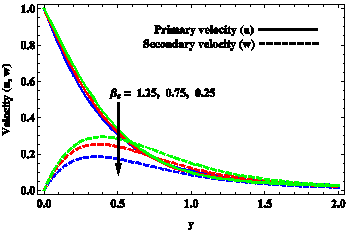(a) $n=3$

## 2b.png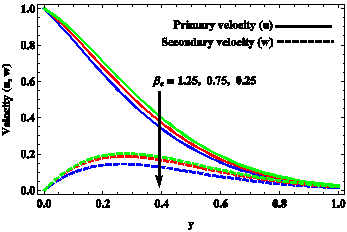(b) $n=15$

Figure 2. Velocity distributions for (a) $n<{{X}_{2}}$ and (b) $n>{{X}_{2}}$, when  ${{\beta }_{i}}=0.5,\,\,{{K}^{2}}=3,\,\,{{M}^{2}}=9,\,\,{{k}_{1}}=0.3,\,\,{{G}_{T}}=4,$  ${{G}_{C}}=5,\,\,{{P}_{r}}=0.71,\,\,{{S}_{c}}=0.22,\,\,\,\phi =1\,\,\text{and}\,{{K}_{1}}=0.2.$

## 3a.png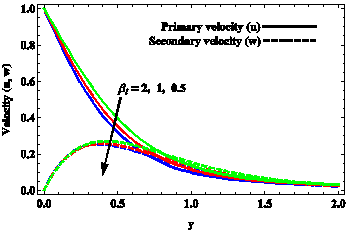(a) $n=3$

## 3b.png(b) $n=15$

Figure 3. Velocity distributions for (a) $n<{{X}_{2}}$ and (b) $n>{{X}_{2}}$ when  ${{\beta }_{e}}=0.75,\,\,{{K}^{2}}=3,\,\,{{M}^{2}}=9,\,\,{{k}_{1}}=0.3,\,\,{{G}_{T}}=4,$ ${{G}_{C}}=5,\,\,{{P}_{r}}=0.71,\,\,{{S}_{c}}=0.22,\,\,\,\phi =1\,\,\text{and}\,{{K}_{1}}=0.2.$

## 4a.png(a) $n=3$

## 4b.png(b) $n=15$

Figure 4. Velocity distributions for (a) $n<{{X}_{2}}$ and (b) $n>{{X}_{2}}$ when  ${{\beta }_{e}}=0.75,\,\,{{\beta }_{i}}=0.5,\,\,{{M}^{2}}=9,\,\,{{k}_{1}}=0.3,\,\,{{G}_{T}}=4,$ ${{G}_{C}}=5,\,\,{{P}_{r}}=0.71,\,\,{{S}_{c}}=0.22,\,\,\,\phi =1\,\,\text{and}\,{{K}_{1}}=0.2.$

## 5a.png(a) $n=3$

## 5b.png(b) $n=15$

Figure 5. Velocity distributions for (a) $n<{{X}_{2}}$ and (b) $n>{{X}_{2}}$ when  ${{\beta }_{e}}=0.75,\,\,{{\beta }_{i}}=0.5,\,\,{{K}^{2}}=3,\,\,{{k}_{1}}=0.3,\,\,{{G}_{T}}=4,$ ${{G}_{C}}=5,\,\,{{P}_{r}}=0.71,\,\,{{S}_{c}}=0.22,\,\,\,\phi =1\,\,\text{and}\,{{K}_{1}}=0.2.$

## 6a.png(a) $n=3$

## 6b.png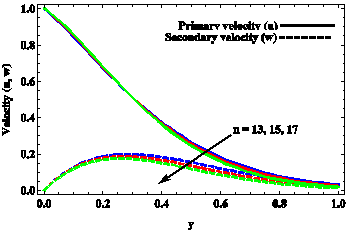(b) $n=15$

Figure 6. Velocity distributions for (a) $n<{{X}_{2}}$ and (b) $n>{{X}_{2}}$ when  ${{\beta }_{e}}=0.75,\,\,{{\beta }_{i}}=0.5,\,\,\,{{K}^{2}}=3,\,\,\,{{M}^{2}}=9,\,\,{{G}_{T}}=4,$ ${{G}_{C}}=5,\,\,{{P}_{r}}=0.71,\,\,{{S}_{c}}=0.22,\,\,\,\phi =1\,\,\text{and}\,{{K}_{1}}=0.2.$

## 7a.png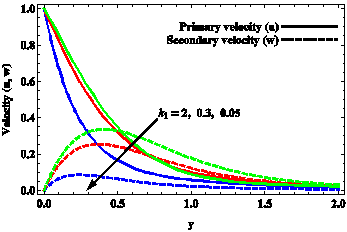(a) $n=3$

## 7b.png(b) $n=15$

Figure 7. Velocity distributions for (a) $n<{{X}_{2}}$ and (b) $n>{{X}_{2}}$ when  ${{\beta }_{e}}=0.75,\,\,{{\beta }_{i}}=0.5,\,\,\,{{K}^{2}}=3,\,\,\,{{M}^{2}}=9,\,\,{{G}_{T}}=4,$ ${{G}_{C}}=5,\,\,{{P}_{r}}=0.71,\,\,{{S}_{c}}=0.22,\,\,\,\phi =1\,\,\text{and}\,{{K}_{1}}=0.2.$

## 8a.png(a) $n=3$

## 8b.png(b) $n=15$

Figure 8. Velocity distributions for (a) $n<{{X}_{2}}$ and (b) $n>{{X}_{2}}$ when  ${{\beta }_{e}}=0.75,\,\,{{\beta }_{i}}=0.5,\,\,\,{{K}^{2}}=3,\,\,\,{{M}^{2}}=9,\,{{k}_{1}}=0.3,$ ${{G}_{C}}=5,\,\,{{P}_{r}}=0.71,\,\,{{S}_{c}}=0.22,\,\,\,\phi =1\,\,\text{and}\,\,{{K}_{1}}=0.2.$

## 9a.png(a) $n=3$

## 9b.png(b) $n=15$

Figure 9. Velocity distributions for (a) $n<{{X}_{2}}$ and (b) $n>{{X}_{2}}$ when  ${{\beta }_{e}}=0.75,\,\,{{\beta }_{i}}=0.5,\,\,\,{{K}^{2}}=3,\,\,\,{{M}^{2}}=9,\,\,{{k}_{1}}=0.3,$ ${{G}_{T}}=4,\,\,{{P}_{r}}=0.71,\,\,{{S}_{c}}=0.22,\,\,\phi =1\,\,\text{and}\,\,{{K}_{1}}=0.2.$

## 10a.png(a) $n=3$

## 10b.png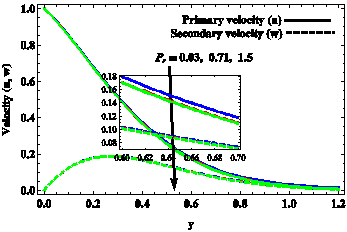(b) $n=15$

Figure 10. Velocity distributions for (a) $n<{{X}_{2}}$ and (b) $n>{{X}_{2}}$ when  ${{\beta }_{e}}=0.75,\,\,{{\beta }_{i}}=0.5,\,\,\,{{K}^{2}}=3,\,\,\,{{M}^{2}}=9,\,\,{{k}_{1}}=0.3,$ ${{G}_{T}}=4,\,\,{{G}_{C}}=5,\,\,\,{{S}_{c}}=0.22,\,\,\,\phi =1\,\,\text{and}\,\,{{K}_{1}}=0.2.$

## 11a.png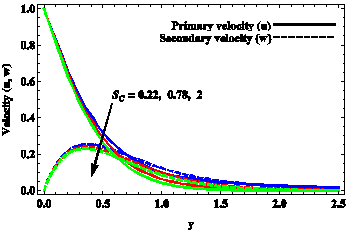(a) $n=3$

## 11b.png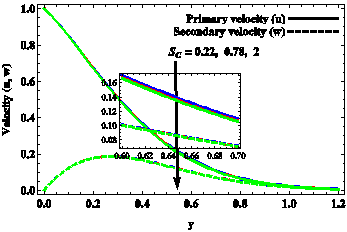(b) $n=15$

Figure 11. Velocity distributions for (a) $n<{{X}_{2}}$ and (b) $n>{{X}_{2}}$ when  ${{\beta }_{e}}=0.75,\,\,{{\beta }_{i}}=0.5,\,\,\,{{K}^{2}}=3,\,\,\,{{M}^{2}}=9,\,\,{{k}_{1}}=0.3,$ ${{G}_{T}}=4,\,\,{{G}_{C}}=5,\,\,\,{{P}_{r}}=0.71\,,\,\,\,\phi =1\,\,\,\text{and}\,\,{{K}_{1}}=0.2.$

## 12a.png(a) $n=3$

## 12ba.png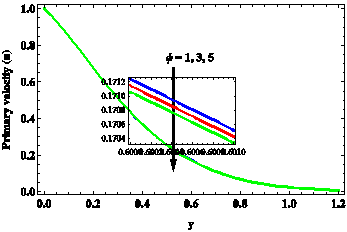## 12bb.png(b) $n=15$

Figure 12. Velocity distributions for (a) $n<{{X}_{2}}$ and (b) $n>{{X}_{2}}$ when  ${{\beta }_{e}}=0.75,\,\,{{\beta }_{i}}=0.5,\,\,\,{{K}^{2}}=3,\,\,\,{{M}^{2}}=9,\,\,{{k}_{1}}=0.3,$ ${{G}_{T}}=4,\,\,{{G}_{C}}=5,\,\,{{P}_{r}}=0.71,\,\,{{S}_{c}}=0.22\,\,\text{and}\,\,{{K}_{1}}=0.2.$

## 13a.png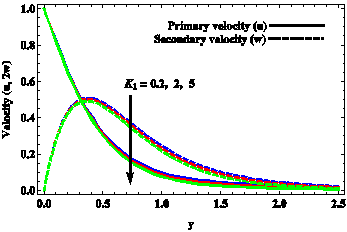(a) $n=3$

## 13ba.png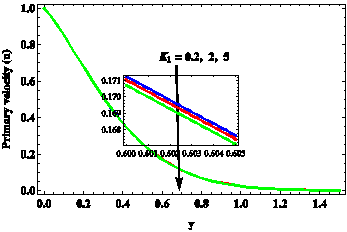## 13bb.png(b) $n=15$

Figure 13. Velocity distributions for (a) $n<{{X}_{2}}$ and (b) $n>{{X}_{2}}$ when  ${{\beta }_{e}}=0.75,\,\,{{\beta }_{i}}=0.5,\,\,\,{{K}^{2}}=3,\,\,\,{{M}^{2}}=9,\,\,{{k}_{1}}=0.3,$ ${{G}_{T}}=4,\,\,{{G}_{C}}=5,\,\,{{P}_{r}}=0.71\,,\,\,\,{{S}_{c}}=0.22\,\,\,\,\text{and}\,\,\phi =1\,.$

## 14.pngFigure 14. Temperature distributions when $\phi =1$.

## 15.png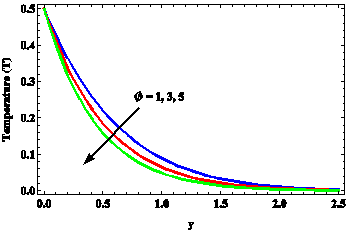Figure 15. Temperature distributions when ${{P}_{r}}=0.71$.

## 16.png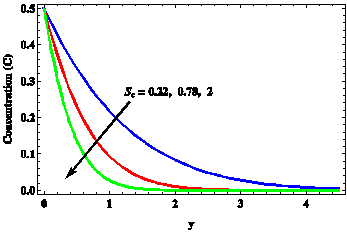Figure 16. Concentration distributions when ${{K}_{1}}=0.2$.

## 17.pngFigure 17. Concentration distributions when ${{S}_{c}}=0.22$.

Table 2. Skin friction distributions for (a) $n<{{X}_{2}}$ and (b) $n>{{X}_{2}}$ when  ${{K}^{2}}=3,\,\,{{M}^{2}}=9,\,\,{{k}_{1}}=0.3,\,\,{{G}_{T}}=4,\,\,{{G}_{C}}=5,$ ${{P}_{r}}=0.71,\,\,{{S}_{c}}=0.22,\,\,\,\phi =1\,\,\text{and}\,\,{{K}_{1}}=0.2.$

(a) $n=3$

 ${{\beta }_{i}}$↓ ${{\beta }_{e}}$→ 0.25 0.75 1.25 $-{{\tau }_{x}}$ 0.5 2.174 1.8478 1.5912 1 2.0394 1.6477 1.4088 2 1.8251 1.3718 1.1585 ${{\tau }_{y}}$ 0.5 1.4093 1.7833 1.9311 1 1.4076 1.7055 1.8166 2 1.4194 1.6615 1.7516

(b) $n=15$

 ${{\beta }_{i}}$↓${{\beta }_{e}}$→ 0.25 0.75 1.25 $-{{\tau }_{x}}$ 0.5 1.5973 1.2037 0.9145 1 1.4660 1.0301 0.7787 2 1.2592 0.7895 0.5792 ${{\tau }_{y}}$ 0.5 1.3332 1.6381 1.7131 1 1.3100 1.5142 1.5514 2 1.2835 1.4021 1.4186

Table 3. Skin friction distributions for (a) $n<{{X}_{2}}$and (b)  $n>{{X}_{2}}$when ${{\beta }_{e}}=0.75,\,\,\,{{\beta }_{i}}=0.5,\,{{k}_{1}}=0.3,\,\,{{G}_{T}}=4,\,\,{{G}_{C}}=5,$ ${{P}_{r}}=0.71,\,\,\,{{S}_{c}}=0.22,\,\,\,\phi =1\,\,\text{and}\,\,{{K}_{1}}=0.2.$

(a) $n=3$

 ${{K}^{2}}$↓ ${{M}^{2}}$→ 9 16 25 $-{{\tau }_{x}}$ 1 1.9535 2.9577 3.9638 3 2.1740 3.0963 4.058 5 2.4651 3.2837 4.1833 ${{\tau }_{y}}$ 1 0.7157 0.7384 0.7845 3 1.4093 1.2872 1.2298 5 1.9883 1.7828 1.6489

(b) $n=15$

 ${{K}^{2}}$↓${{M}^{2}}$→ 9 16 25 $-{{\tau }_{x}}$ 1 1.4725 2.4425 3.4804 3 1.5973 2.5515 3.571 5 1.8008 2.7153 3.6977 ${{\tau }_{y}}$ 1 0.6492 0.7384 0.8182 3 1.3332 1.3098 1.2905 5 1.9790 1.8521 1.7423

Table 4. Skin friction distributions for (a) $n<{{X}_{2}}$ and (b) $n>{{X}_{2}}$ when $\,{{\beta }_{e}}=0.75,\,\,{{\beta }_{i}}=0.5,\,\,{{K}^{2}}=3,\,\,{{M}^{2}}=9,\,\,{{G}_{T}}=4,$ ${{G}_{C}}=5,\,\,{{P}_{r}}=0.71,\,\,{{S}_{c}}=0.22,\,\,\phi =1\,\,\text{and}\,\,{{K}_{1}}=0.2.$

(a) $n=3$

 ${{k}_{1}}$↓  $n$→ 3 5 7 $-{{\tau }_{x}}$ 0.05 4.0667 4.1928 4.1357 0.3 1.8478 1.9939 1.88 2 1.3993 1.5242 1.3721 ${{\tau }_{y}}$ 0.05 1.0027 0.988 0.9991 0.3 1.7833 1.7473 1.7526 2 2.0953 2.0565 2.0395

(b) $n=15$

 ${{k}_{1}}$$n$→ 13 15 17 $-{{\tau }_{x}}$ 0.05 3.7337 3.5847 3.4380 0.3 1.3584 1.2037 1.0627 2 0.8251 0.6776 0.5462 ${{\tau }_{y}}$ 0.05 1.0421 1.0499 1.0541 0.3 1.6778 1.6381 1.5974 2 1.8693 1.8056 1.745

Table 5. Skin friction distributions for (a) $n<{{X}_{2}}$ and (b) $n>{{X}_{2}}$ when $\,{{\beta }_{e}}=0.75,\,\,{{\beta }_{i}}=0.5,\,\,{{K}^{2}}=3,\,\,{{M}^{2}}=9,\,\,{{k}_{1}}=0.3,$ ${{P}_{r}}=0.71,\,\,{{S}_{c}}=0.22,\,\,\phi =1\,\,\text{and}\,\,{{K}_{1}}=0.2.$

(a) $n=3$

 ${{G}_{C}}$↓${{G}_{T}}$→ 2 4 6 $-{{\tau }_{x}}$ 3 2.2692 2.0746 1.8800 5 2.0423 1.8478 1.6532 7 1.8155 1.6209 1.4263 ${{\tau }_{y}}$ 3 1.6747 1.7198 1.7650 5 1.7382 1.7833 1.8285 7 1.8017 1.8468 1.892

(b) $n=15$

 ${{G}_{C}}$↓ ${{G}_{T}}$→ 2 4 6 $-{{\tau }_{x}}$ 3 1.2591 1.2343 1.2094 5 1.2285 1.2037 1.1788 7 1.1978 1.173 1.1482 ${{\tau }_{y}}$ 3 1.6328 1.6349 1.6370 5 1.6360 1.6381 1.6402 7 1.6392 1.6413 1.6434

Table 6. Skin friction distributions for (a) $n<{{X}_{2}}$ and (b) $n>{{X}_{2}}$ when ${{\beta }_{e}}=0.75,\,\,{{\beta }_{i}}=0.5,\,\,{{K}^{2}}=3,\,\,{{M}^{2}}=9,\,\,{{k}_{1}}=0.3,$ ${{G}_{T}}=4,\,\,{{G}_{C}}=5,\,\,\phi =1\,\,\text{and}\,\,{{K}_{1}}=0.2.$

(a) $n=3$

 ${{S}_{c}}$↓  ${{P}_{r}}$→ 0.03 0.71 1.5 $-{{\tau }_{x}}$ 0.22 1.7366 1.8478 1.8937 0.78 1.8100 1.9212 1.9672 2 1.8831 1.9943 2.0402 ${{\tau }_{y}}$ 0.22 1.8545 1.7833 1.7615 0.78 1.8109 1.7398 1.7179 2 1.7764 1.7052 1.6833

(b) $n=15$

 ${{S}_{c}}$↓  ${{P}_{r}}$→ 0.03 0.71 1.5 $-{{\tau }_{x}}$ 0.22 1.1793 1.2037 1.2116 0.78 1.1944 1.2188 1.2267 2 1.2069 1.2313 1.2392 ${{\tau }_{y}}$ 0.22 1.6435 1.6381 1.6368 0.78 1.6405 1.6351 1.6339 2 1.6387 1.6332 1.632

Table 7. Skin friction distributions for (a) $n<{{X}_{2}}$ and (b) $n>{{X}_{2}}$ when ${{\beta }_{e}}=0.75,\,\,\,{{\beta }_{i}}=0.5,\,\,{{K}^{2}}=3,\,\,{{M}^{2}}=9,\,\,{{k}_{1}}=0.3,$ ${{G}_{T}}=4,\,\,{{G}_{C}}=5,\,\,{{P}_{r}}=0.71\,\,\text{and}\,\,{{S}_{c}}=0.22.$

(a) $n=3$

 ${{K}_{1}}$↓  $\phi$→ 1 3 5 $-{{\tau }_{x}}$ 0.2 1.8478 1.8709 1.8889 2 1.8689 1.8921 1.9101 5 1.8965 1.9196 1.9377 ${{\tau }_{y}}$ 0.2 1.7833 1.7749 1.7668 2 1.7723 1.7639 1.7558 5 1.7582 1.7497 1.7417

(b) $n=15$

 ${{K}_{1}}$↓  $\phi$→ 1 3 5 $-{{\tau }_{x}}$ 0.2 1.2037 1.2058 1.2087 2 1.2045 1.2066 1.2095 5 1.2058 1.2079 1.2108 ${{\tau }_{y}}$ 0.2 1.6381 1.6416 1.6431 2 1.638 1.6414 1.643 5 1.6377 1.6412 1.6428

Table 8. Nusselt number

 $-Nu$ ${{P}_{r}}$↓ $\phi$→ 1 3 5 0.03 0.1601 0.1987 0.2321 0.71 0.7791 0.9669 1.1294 1.5 1.1324 1.4054 1.6416

Table 9. Sherwood number

 $-Sh$ ${{S}_{c}}$↓${{K}_{1}}$→ 0.2 2 5 0.22 0.3865 0.4880 0.6286 0.78 0.7279 0.9190 1.1837 2 1.1656 1.4716 1.8955
7. Conclusions

A mathematical analysis has been presented for unsteady MHD free convection flow of an incompressible, chemically reacting, electrically and thermally conducting fluid past an oscillating vertical plate embedded in a fluid saturated porous medium in rotating system taking Hall and ion-slip currents into account. When the natural frequency due to rotation and Hall current is different from the frequency of oscillations, the significant flow features are summarized below:

Ion-slip, thermal buoyancy force, concentration buoyancy force, thermal diffusion and chemical molecular diffusion have tendency to enhance fluid velocity in the primary flow direction whereas Coriolis force, Lorentz force, heat absorption and chemical reaction have reverse tendency on it. Hall current, Coriolis force, thermal buoyancy force, concentration buoyancy force, thermal diffusion and chemical molecular diffusion have tendency to enhance fluid velocity in the secondary flow direction whereas Darcian drag force, oscillations, heat absorption and chemical reaction have reverse tendency on it. When the natural frequency is greater than the frequency of oscillations, oscillations has tendency to reduce fluid velocity in the primary flow direction whereas Hall current has tendency to reduce it in the region away from the plate. Ion-slip has tendency to enhance fluid velocity in the primary flow direction whereas Lorentz force has reverse tendency on it. When the natural frequency is smaller than the frequency of oscillations, Darcian drag force has tendency to reduce fluid velocity in the primary flow direction while ion-slip has tendency to reduce fluid velocity in the secondary flow direction. Oscillations has tendency to enhance fluid velocity in the primary flow direction in the vicinity of the plate while this tendency is reversed in the region away from the plate. Lorentz force has the same effect as that of oscillations on the fluid velocity in the secondary flow direction.

Coriolis force, Lorentz force, Darcian drag force, heat absorption and chemical reaction have tendency to enhance skin friction in the primary flow direction whereas Hall current, ion-slip, oscillations, thermal buoyancy force, concentration buoyancy force, thermal diffusion and chemical molecular diffusion have reverse tendency on it. Hall current, Coriolis force, Lorentz force, thermal buoyancy force, concentration buoyancy force, thermal diffusion and chemical molecular diffusion have tendency to enhance skin friction in the secondary flow direction whereas Darcian drag force and chemical reaction have reverse tendency on it. When the natural frequency is greater than the frequency of oscillations, heat absorption has tendency to reduce skin friction in the primary flow direction. When the natural frequency is smaller than the frequency of oscillations, heat absorption has tendency to enhance skin friction in the secondary flow direction.

Acknowledgment

Authors P. Rohidas [File No: F1-17.1/2016-17/RGNF-2015-17-SC-KAR-10391/(SA-III/Website)] and S. G. Begum [File No: F1-17.1/2014-15/MANF-2014-15-MUS-KAR35378/(SA-III/Website)] are thankful to University Grant Commission (UGC), New Delhi (India) for providing Junior Research Fellowship to carry out this research work.

Nomenclature
 ${{B}_{0}}$ applied magnetic field $(T)$ $C$ ${C}'$ Non-dimensional species concentration species concentration $(mol/{{m}^{3}})$ ${{C}_{p}}$ specific heat at constant pressure $(J/kg.K)$ D chemical molecular diffusivity $({{m}^{2}}/s)$ $g$ acceleration due to gravity $\left( m/{{s}^{2}} \right)$ $\vec{g}$ gravitational field vector ${{G}_{C}}$ solutal Grashof number ${{G}_{T}}$ thermal Grashof number $h$ characteristic length $(m)$ ${\vec{J}}'$ current density vector $k$ thermal conductivity of the fluid $(W/m.K)$ ${k}'$ permeability $({{m}^{2}})$ $K$ rotation parameter ${{k}_{1}}$ permeability parameter ${{K}_{1}}$ chemical reaction parameter $M$ magnetic parameter $n$ frequency parameter ${{P}_{r}}$ Prandtl number ${{Q}_{0}}$ heat absorption coefficient $(W/{{m}^{2}}.K)$ $s$ Laplace parameter ${{S}_{c}}$ Schmdit number $t$ non-dimensional time ${t}'$ time $(s)$ ${T}'$ fluid temperature $(K)$ $u$ non-dimensional velocity in $x$-direction ${{U}_{0}}$ characteristic velocity $(m/s)$ $w$ non-dimensional velocity in $z$-direction $y$ non-dimensional coordinate normal to the plate

Greek symbols

 $\varepsilon$ constant ${{\beta }_{C}}$ volumetric coefficient of  species concentration  expansion $({{K}^{-1}})$ ${{\beta }_{e}}$ Hall current parameter ${{\beta }_{i}}$ ion-slip parameter ${{\beta }_{T}}$ volumetric coefficient of thermal expansion ${{\eta }_{m}}$ magnetic diffusivity $({{m}^{2}}/s)$ $\upsilon$ kinematic viscosity $({{m}^{2}}/s)$ ${\omega }'$ frequency of oscillations $\left( 1/s \right)$ $\phi$ heat absorption parameter $\rho$ fluid density $(kg/{{m}^{3}})$ $\sigma$ electrical conductivity $(S/m)$ ${{\tau }_{x}}$ skin friction component in $x$-direction ${{\tau }_{z}}$ skin friction component in $z$-direction $\theta$ non-dimensional fluid temperature

Subscripts

 $w$ conditions at the plate $\infty$ conditions in the free stream
References

 Abo-Eldahab E.M., Aziz M.A.E. (2000). Hall and ion-slip effects on MHD free convective heat generating flow past a semi-infinite vertical flat plate, Physica Scripta, Vol. 61, No. 3, pp. 344-348.

 Ibrahim F.S., Hassanien I.A., Bakr A.A. (2004). Unsteady magnetohydrodynamic micropolar fluid flow and heat transfer over a vertical porous plate through a porous medium in the presence of thermal and mass diffusion with a constant heat source, Canad. J. Phys, Vol. 82, No. 10, pp. 775-790. DOI: 10.1139/P04-021

 Chaudhary R.C., Jain A. (2007). Combined heat and mass transfer effects on MHD free convection flow past an oscillating plate embedded in porous medium, Rom. J. Phys, Vol. 52, No. 5-7, pp. 505-524.

 Postelnicu A. (2006). Influence of chemical reaction on heat and mass transfer by natural convection from vertical surfaces in porous media considering Soret and Dufour effects, Heat and Mass Transfer, Vol. 43, No. 6, pp. 595-602. DOI: 10.1007/s00231-006-0132-8

 Mbeledogu I.U., Ogulu A. (2007). Heat and mass transfer of an unsteady MHD natural convection flow of a rotating fluid past a vertical porous flat plate in the presence of radiative heat transfer, Int. J. Heat Mass Transfer, Vol. 50, No. 9-10, pp. 1902-1908. DOI: 10.1016/j.ijheatmasstransfer.2006.10.016

 Beg O.A., Bakier A.Y., Prasad V.R. (2009). Numerical study of free convection magnetohydrodynamic heat and mass transfer from a stretching surface to a saturated porous medium with Soret and Dufour effects, Comp. Materials Sci, Vol. 46, No. 1, pp. 57-65. DOI: 10.1016/j.commatsci.2009.02.004

 Ghosh S.K., Beg O.A., Zueco J. (2010). Hydromagnetic free convection flow with induced magnetic field effects, Meccanica, Vol. 45, No. 2, pp. 175-185. DOI: 10.1007/s11012-009-9235-x

 Makinde O.D. (2010). Similarity solution of hydromagnetic heat and mass transfer over a vertical plate with a convective surface boundary condition, Int. J. Phy. Sci, Vol. 5, No. 6, pp. 700-710.

 Rahman M.M., Salahuddin K.M. (2010). Study of hydromagnetic heat and mass transfer flow over an inclined heated surface with variable viscosity and electric conductivity, Comm. Nonlinear Sci. Num. Simu., Vol. 15, No. 8, pp. 2073-2085. DOI: 10.1016/j.cnsns.2009.08.012

 Chamkha A.J. (2010). Aly A.M.MHD free convection flow of a nanofluid past a vertical plate in the presence of heat generation or absorption effects, Chem. Eng. Comm., Vol. 198, No. 3, pp. 425-441. DOI: 10.1080/00986445.2010.520232

 Seth G.S., Ansari M.S., Nandkeolyar R. (2011). MHD natural convection flow with radiative heat transfer past an impulsively moving plate with ramped wall temperature, Heat Mass Transfer, Vol. 47, No. 5, pp. 551-561. DOI: 10.1007/s00231-010-0740-1

 Seth G.S., Sarkar S., Hussain S.M. (2014). Effects of hall current, radiation and rotation on natural convection heat and mass transfer flow past a moving vertical plate, Ain Shams Eng. J, Vol. 5, No. 2, pp. 489-503. DOI: 10.1016/j.asej.2013.09.014

 Seth G.S., Tripathi R., Sharma R. (2015). Natural convection flow past an exponentially accelerated vertical ramped temperature plate with Hall effects and heat absorption, Int. J. Heat Tech., Vol. 33, No. 3, pp. 139-144. DOI: 10.18280/ijht.330321

 Das K. (2011). Effect of chemical reaction and thermal radiation on heat and mass transfer flow of MHD micropolar fluid in a rotating frame of reference, Int. J. Heat Mass Transfer, Vol. 54, No. 15-16, pp. 3505-3513. DOI: 10.1016/j.ijheatmasstransfer.2011.03.035

 Khan I., Fakhar K., Shafie S. (2011). Magnetohydrodynamic free convection flow past an oscillating plate embedded in a porous medium, J. Phys. Soc. Japan, Vol. 80, No. 10, 104401 (10 Pages). DOI: 10.1143/JPSJ.80.104401

 Prasad V.R., Vasu B., Beg O.A. (2011). Thermo-diffusion and diffusion-thermo effects on MHD free convection flow past a vertical porous plate embedded in a non-Darcian porous medium, Chem. Eng. J., Vol. 173, No. 2, pp. 598-606. DOI: 10.1016/j.cej.2011.08.009

 Deka R.K., Paul A., Chaliha A. (2014). Transient free convection flow past an accelerated vertical cylinder in a rotating fluid, Ain Shams Eng. J., Vol. 5, No. 2, pp. 505-513. DOI: 10.1016/j.asej.2013.10.002

 Narahari M., Debnath L. (2013). Unsteady magnetohydrodynamic free convection flow past an accelerated vertical plate with constant heat flux and heat generation or absorption, ZAMM, Vol. 93, No. 1, pp. 38-49. DOI: 10.1002/zamm.201200008

 Hossain M.D., Samad M.A., Alam M.M. (2015). MHD free convection and mass transfer flow through a vertical oscillatory porous plate with Hall, ion-slip currents and heat source in a rotating system, Procedia Eng, Vol. 105, pp. 56-63. DOI: 10.1016/j.proeng.2015.05.006

 Balamurugan K.S., Ramaprasad J.L., Varma S.V.K. (2015). Unsteady MHD free convective flow past a moving vertical plate with time dependent suction and chemical reaction in a slip flow regime, Procedia Eng., Vol. 127, pp. 516-523. DOI: 10.1016/j.proeng.2015.11.338

 Swetha R., Reddy G.V., Varma S.V.K. (2015). Diffusion-thermo and radiation effects on MHD free convection flow of a chemically reacting fluid past an oscillating plate embedded in porous medium, Procedia Eng, Vol. 127, pp. 553-560. DOI: 10.1016/j.proeng.2015.11.344

 Reddy Y.S., Varma S.V.K., Balamurugan K.S., Ramaprasad J.L. (2015). Chemical reaction and radiation absorption effects on hydromagnetic free convection flow past a vertical plate with constant mass flux, Far East J. Math. Sci., Vol. 98, No. 2, pp. 133-149. DOI: 10.17654/FJMSSep2015_133_149

 Seth G.S., Sarkar S. (2015). Hydromagnetic natural convection flow with induced magnetic field and nth order chemical reaction of a heat absorbing fluid past an impulsively moving vertical plate with ramped temperature, Bulg. Chem. Com., Vol. 47, No. 1, pp. 66-79.

 Butt A.S., Ali A. (2016). Entropy generation effects in a hydromagnetic free convection flow past a vertical oscillating plate, J. Appl. Mech. Tech. Phy, Vol. 57, No. 1, pp. 27-37. DOI: 10.1134/S0021894416010053

 Raptis A.A., Singh A.K. (1985). Rotation effects on MHD free-convection flow past an accelerated vertical plate, Mech. Res. Commun., Vol. 12, No. 1, pp. 31-40. DOI: 10.1016/0093-6413(85)90032-1

 Kythe P.K., Puri P. (1988). Unsteady MHD free convection flows on a porous plate with time-dependent heating in a rotating medium, Astrophys. Space Sci., Vol. 143, No. 1, pp. 51-62. DOI: 10.1007/BF00636754

 Tokis J.N. (1988). Free convection and mass transfer effects on the magnetohydrodynamic flows near a moving plate in a rotating medium, Astrophys. Space Sci., Vol. 144, No. 1-2, pp. 291-301.

 Nanousis N. (1992). Thermal diffusion effects on MHD free convective and mass transfer flow past a moving infinite vertical plate in a rotating fluid, Astrophys. Space Sci., Vol. 191, No. 2, pp. 313-322. DOI: 10.1007/BF00644779

 Cramer K.R., Pai S.I. (1973). Magnetofluid-Dynamics for Engineers and Applied Physicists, McGraw-Hill, New York.

 Cowling T.G. (1957). Magnetohydrodynamics, Interscience, New York.

 Meyer R.C. (1958). On reducing aerodynamic heat-transfer rates by magnetohydrodynamic technique, J. Aerospace Sci., Vol. 25, No. 9, pp. 561–566. DOI: 10.2514/8.7781

 Sutton G., Sherman A. (1965). Engineering Magnetohydrodynamics, McGraw-Hill, New York.